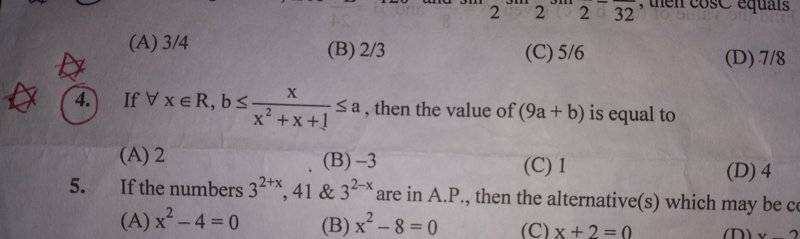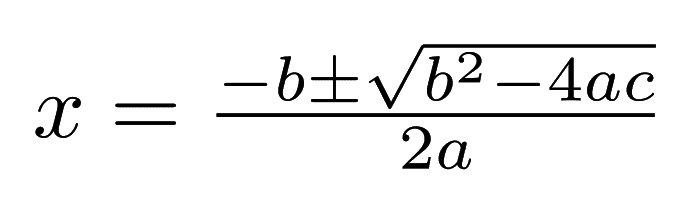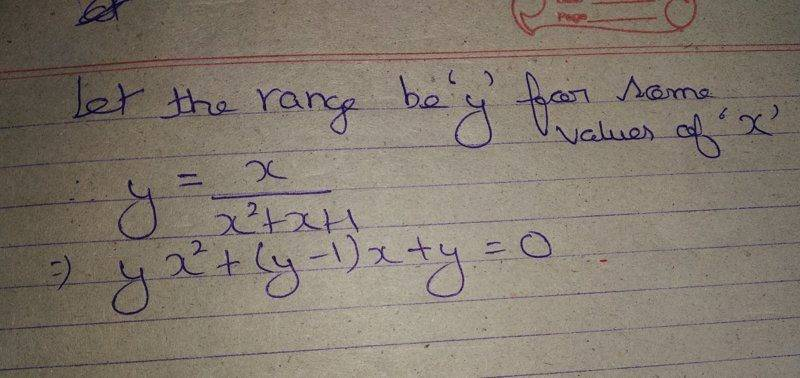# Need help in solving this question about a rational inequality

• navneet9431
In summary, the conversation discusses finding the range of the function x/(x^2+x+1) and the application of the condition that Determinant(D)>=0 to solve the problem. The function is bounded by a and b, approaches zero for large or very negative x, and has both a maximum and minimum value. This problem can be solved using techniques from calculus to find the two extreme points.

Gold Member

## Homework StatementGo through question number 4

## Homework Equations## The Attempt at a Solution

See basically the question is asking us to find the range of the given function x/(x^2+x+1).
So,I began solving it this way...I am stuck at this step.
I asked my friend for a hint and he told me to apply the condition that Determinant(D)>=0.
But,I am unable to understand why D>=0?
I will be thankful for help!

#### Attachments

Last edited by a moderator:
The function that is bounded by a and b is ##f(x) = \frac x {x^2 + x + 1}##. For large x or very negative x, f(x) approaches zero. The function is continuous everywhere, and has both a maximum and minumum value. You have posted this question in the Precalc section, but the problem is one that can be solved using techniques from calculus, to find the two extreme points.

BTW, in your thread title, you described this as a problem about a polynomial equation, which it isn't. I've edited your title so that it is about a rational inequality.

•jedishrfu